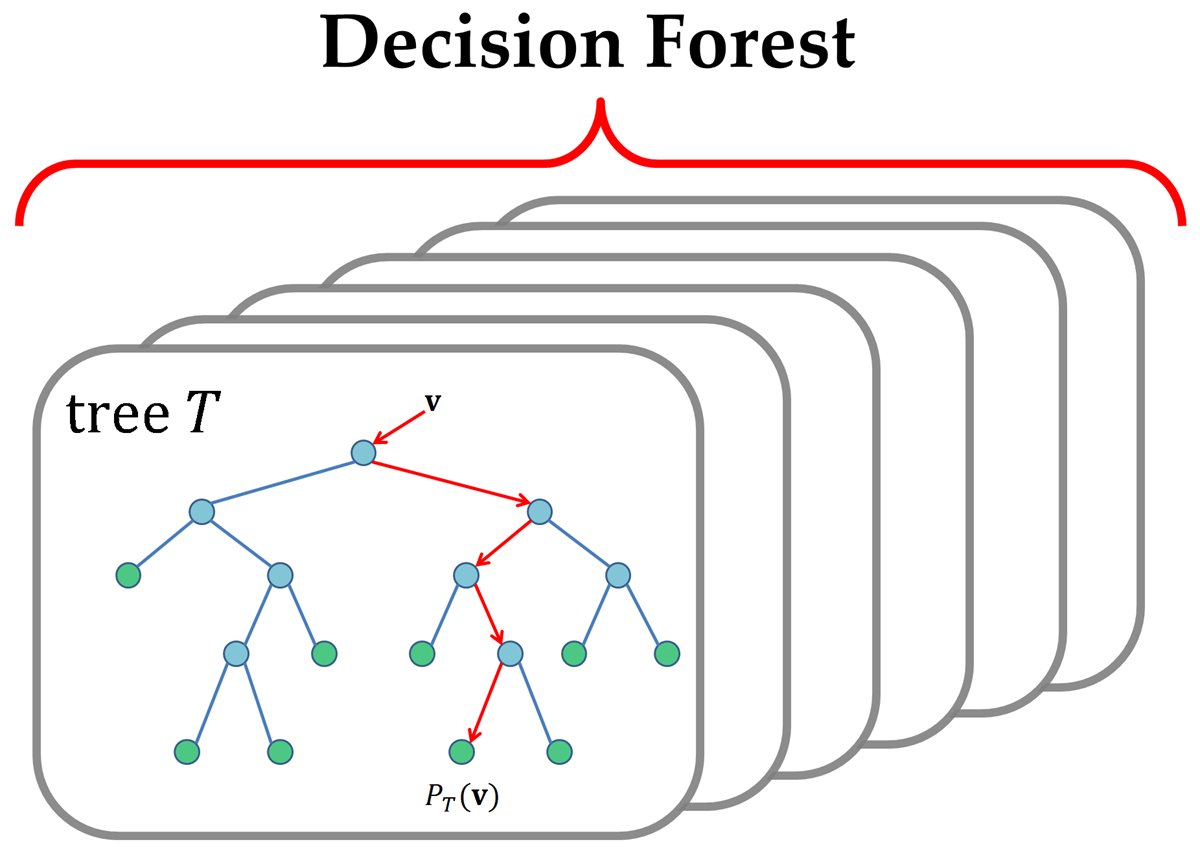×

Search anything:

# Random Decision Forest

#### Machine Learning (ML) random decision forestGet this book -> Problems on Array: For Interviews and Competitive Programming

Random forests or random decision forests are an ensemble learning method for classification, regression and other tasks that operates by constructing a multitude of decision trees at training time.

### Inituition

Imagine you are at a party, and you have to guess the number of jellybeans in a jar full of them.A common way of approaching this, something which most people will do, is to estimate the number of jellybeans to the best of your ability.

Alternatively, a better way to approach this problem is to wait for people to make their guesses, and calculate the average of everyone's guesses. This way, your choice is no longer limited to your own perception. Since you are now including everyone's estimates, it is almost as if multiple people are working for your guess, instead of one. Statistically, you now have a much higher probability of guessing close to the actual number, and makes your guess much likely to do well.

This approach is an application of ensemble learning.

## Ensemble Learning

In machine learning, ensemble methods use multiple learning algorithms to obtain better predictive performance than could be obtained from any of the constituent learning algorithms alone.

The first method of estimating the number of jellybeans alone, is analogous to the Decision Tree algorithm, where one decision tree is used to fit the model. The predictions made in this case may be biased or susceptible to little changes. The alternative method we discussed, ensemble learning, is what we do in the Random Forest algorithm.

## What are Random Forests?

Random forests or random decision forests are an ensemble learning method for classification, regression and other tasks that operates by constructing a multitude of decision trees at training time.Random Forests, for a predefined number N, train N decision trees on the training data, with each tree being independent and random, and have each decision tree make it's own prediction. The final prediction is either the average of all the predictions made by individual trees (Regression), or the most common prediction made by the trees (Classification)

This approach, makes the algorithm much more robust to any anomalies in the dataset, handles any missing values better, and is overall more stable as compared to using a single decision tree.

Random Forest is not only versatile in terms of the kind of problem we are trying to solve, but also whether it is a classification or a regression problem. The only thing that changes is the way we interpret our output

## Pseudocode

Here's a simplified form of how Random Forest works:

STEP 1: Pick any K data points at random from the training set
STEP 2: Build the decision tree associated to these K data points
STEP 3: Repeat STEPS 1 and 2 N times, where N = number of trees.

For making a prediction on the test set, we make each of the decision trees predict the value of Y for the input data point.

## Implementation in scikit-learn

``````from sklearn.ensemble import RandomForestClassifier
rf = RandomForestClassifier(n_estimators=100)
rf.fit(X_train,y_train)
predictions = clf.predict(X_test)
``````

As we can see, using Random Forests in Python is incredibly simple with scikit-learn, and it can be used just as simply as any other algorithm.

## Important Hyperparameters

• n_estimators: This indicates the number of decision tress we intend to use. Generally, the higher the number of trees, the more accurate will our model be.
• random_state: Since Random Forest uses a considerable degree of randomness in it's approach, our predictions may vary with different random states. We use this for consistency.
• max_features: This helps in increasing the accuracy of our model. It is the maximum number of features to consider when splitting a node.

• Random Forests can handle missing values and anomalies well
• Random Forests are generally good with large datasets
• Random Forests normally prevent over-fitting
• They can handle both Classification and Regression problems well
• Random Forests work well with most problems

## Applications of Random Forests

Random Forests find their applications in a variety of areas,a few notable ones are: